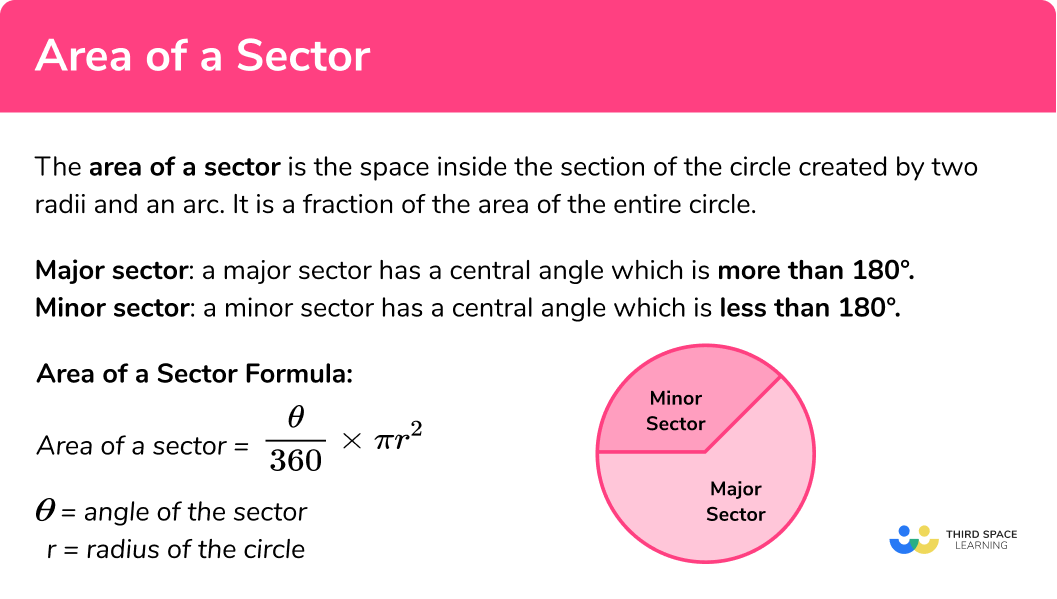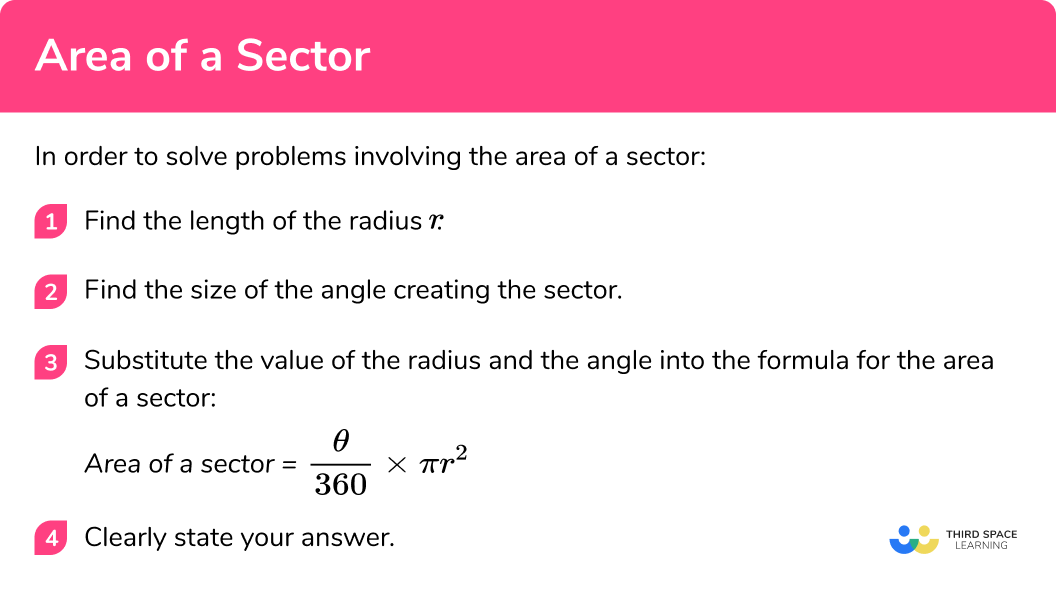# Area Of A Sector

Here we will learn how to calculate the area of a sector including how to identify a sector of a circle, form and use the formula for the area of a sector of a circle and calculate the area of a sector in various scenarios.

There are also area of a sector circle worksheets based on Edexcel, AQA and OCR exam questions, along with further guidance on where to go next if you’re still stuck.

## What is the area of a sector?

The area of a sector is the space inside the section of the circle created by two radii and an arc. It is a fraction of the area of the entire circle.

Major sector: a major sector has a central angle which is more than 180° .
Minor sector: a minor sector has a central angle which is less than 180° .

Area of a sector=\frac{\theta}{360} \times \pi r^{2}

θ = angle of the sector

r = radius of the circle

### What is the area of a sector?## How to find the area of a sector

In order to find the area of a sector you need to be able to find the area of a circle. This is because the area of a sector is part of the total area of the circle.

‘How much’ of the circle is decided by the angle created by the two radii.

The sum of the angle around a point is equal to 360° .

Therefore, the area of a sector is the fraction of the full circle’s area.

The angle is out of 360 or \frac{\theta}{360} , where θ represents the angle, so we can multiply this by the area of the circle to calculate the area of a sector.

NOTE: At GCSE, all angles are measured in degrees. Make sure that your calculator has a small ‘d’ for degrees at the top of the screen rather than an ‘r’ for radians – these are not used until A Level.

Area of a sector formula:

Area of a sector = \frac{\theta}{360} \times \pi r^{2}

θ = angle of the sector

r = radius of the circle

In order to solve problems involving the area of a sector you should follow the below steps:

1. Find the length of the radius \pmb{r}.
2. Find the size of the angle creating the sector.
3. Substitute the value of the radius and the angle into the formula for the area of a sector.

### How to find the area of a sectorArea of a sector is part of our series of lessons to support revision on sector of a circle and circles, sectors and arcs. You may find it helpful to start with the main circles, sectors and arcs lesson for a summary of what to expect, or use the step by step guides below for further detail on individual topics. Other lessons in this series include:

## Area of a sector examples

### Example 1: calculate the area of a sector (quadrant)

Calculate the area of the segment shown below. Give your answer to 3 decimal places.

1. Find the length of the radius.

2Find the size of the angle creating the sector.

Angle = 90° (shown by the symbol of the right angle).

3Substitute the value of the radius and the angle into the formula for the area of a sector.

Area of a sector = \frac{\theta}{360} \times \pi r^{2}

= \frac{90}{360} \times \pi\times 6^{2}

= 9\pi

Area of a sector = {9\pi}cm^2

= 28.274333…cm^2

= 28.274cm^2 (3 d.p.)

### Example 2: calculate the area of a sector (semicircle)

Calculate the area of the semicircle shown below. Give your answer in terms of \pi.

Find the length of the radius.

Find the size of the angle creating the sector

Substitute the value of the radius, the angle into the formula for the area of a sector.

### Example 3: calculate the area of a sector (with an angle given)

Calculate the area of the sector shown below. Give your answer to 3 significant figures.

Find the length of the radius.

Find the size of the angle creating the sector.

Substitute the value of the radius, the angle into the formula for the area of a sector.

### Example 4: calculate the area of a sector (the angle is not given)

Calculate the area of the sector AOB below.

The length of the radius (OB) is 9cm

The length of a chord (AB) is 10cm .

Find the length of the radius

Find the size of the angle creating the sector

Substitute the value of the radius, the angle into the formula for the area of a sector.

## How to solve problems involving an area of a sector

Sometimes you may be given the area of the sector and asked to find a property of the circle such as the radius. In this case you need to ‘reverse’ the process.

1. Clearly state which of the properties you know and do not know (e.g. radius, angle of sector, area of sector).
2. Using the formula for the area of a sector create an equation and solve for the unknown property you have been asked to find.

## Solving problems involving an area of a sector examples

### Example 5: finding the radius given the area of the sector

The sector below has an area of 20cm^2 . Calculate the length of x . Give you answer to 2 decimal places

Clearly state which of the properties you know and do not know (e.g. radius, angle of sector, area of sector).

Using the formula for the area of a sector create an equation and solve for the unknown property you have been asked to find.

### Example 6: finding the angle of a sector given the area of the sector

The sector below has an area of 62cm^2 . Calculate the length of x . Give you answer to 2 decimal places

Clearly state which of the properties you know and do not know (e.g. radius, angle of sector, area of sector).

Using the formula for the area of a sector create an equation and solve for the unknown property you have been asked to find.

### Common misconceptions

• Confusing the segment/sector

A segment is made from a chord whilst a sector will have lines (radii) coming from the origin.

Sectors are a portion of a circle – it can be helpful to think of a sector as a pizza slice.

• Finding the area of the whole circle not the area of the sector

Remember to find the fraction of the circle that makes the sector not just the area of the whole circle.

Use

\frac{\theta}{360} \times \pi r^{2}

Not

\pi r^2

To find the area of a sector

• Incorrect use of the cosine rule

Many mistakes are made when applying other rules within a sector question, such as the cosine rule. Take your time with these parts and regularly ask if your answer makes sense within the context of the question. If you need to review the cosine rule do so before continuing on further.

### Practice area of a sector questions

1. What is the angle of the sector for a quadrant?

90^{\circ}180^{\circ}270^{\circ}360^{\circ}A quadrant is a quarter of a circle. Angles around a point are equal to 360^{\circ} so a quadrant will be a quarter of 360^{\circ} .

360\div4 =90

2. What is the angle of the sector for a semi-circle?

90^{\circ}180^{\circ}270^{\circ}360^{\circ}A semi circle is half a circle. Angles around a point are equal to 360^{\circ} so a half will be half of 360^{\circ} .

360\div2 =180

3. Calculate the area of this sector in terms of pi:9\pi cm^{2}100\pi cm^{2}10\pi cm^{2}25\pi cm^{2}Area of a sector = \frac{\theta}{360} \times \pi r^{2}

\frac{90}{360} \times \pi\times 10^{2} =25\pi

4. Calculate the area of this semi circle in terms of pi157.08 cm^{2}100\pi cm^{2}25\pi cm^{2}50\pi cm^{2}Area of a sector = \frac{\theta}{360} \times \pi r^{2}

\frac{180}{360} \times \pi\times 10^{2 }= 50\pi

5. Calculate the area of this sector in terms of pi150.796 cm^{2}48\pi cm^{2}64\pi cm^{2}8\pi cm^{2}Area of a sector = \frac{\theta}{360} \times \pi r^{2}

\frac{270}{360} \times \pi\times 8^{2} = 48\pi

6. Calculate the area of this sector in terms of pi175.93 cm^{2}56\pi cm^{2}64\pi cm^{2}8\pi cm^{2}Area of a sector = \frac{\theta}{360} \times \pi r^{2}

\frac{315}{360} \times \pi\times 8^{2} = 56\pi

### Area of a sector GCSE questions

1. The diagram shows a sector of a circle with centre O .

The radius of the circle is 13cm .
The angle of the sector is 150^{\circ}.Calculate the area of the sector, give your answer correct to 3 significant figures.

(3 marks)

\frac{150}{360}\times \pi \times 13^2

(1)

221.220…

(1)

221 cm^{2}

(1)

2. The diagram shows a sector of a circle with centre O .

The radius of the circle is 6.5cm .
The acute angle AOB is 70^{\circ}.Calculate the area of the sector, give your answer correct to 3 significant figures.

(3 marks)

\frac{290}{360}\times \pi \times 6.5^2

(1)

106.923…

(1)

107 cm^{2}

(1)

3. The diagram shows a sector of a circle with centre O .

The radius of the circle is 4.9cm .
The acute angle AOB is 60^{\circ}.Calculate the area of the sector, give your answer correct to 2 decimal places.

(3 marks)

\frac{60}{360}\times \pi \times 4.9^2

(1)

12.5716…

(1)

12.57 cm^{2}

(1)

4. Below is a sector of a circle with an area of 5\pi cm^{2} and a radius of 10cm . Find the size of the angle labelled x .(3 marks)

5\pi=\frac{x}{360} \times \pi \times 10^2

Correct attempt to form an equation for the area of the sector

(1)

Attempt to solve equation for x , with two steps completed correctly

(1)

18

(1)

5. The sector below has an area of 30 cm^{2} .

Calculate the length of x
Give you answer to 2 decimal places.(4 marks)

30=\frac{135}{360} \times \pi \times x^2

Correct attempt to form an equation for the area of the sector

(1)

\frac{80}{\pi} or 25.46479… seen

(1)

5.04626 negative solution cannot be the solution

(1)

5.05

(1)

## Learning checklist

You have now learned how to:

• Identify and apply circle definitions and properties, including: centre, radius, chord, diameter, circumference
• Identify and apply circle definitions and properties, including tangent, arc, sector and segment
• Calculate areas of a sector
• Calculate a property of a circle given the area of its sector

## Still stuck?

Prepare your KS4 students for maths GCSEs success with Third Space Learning. Weekly online one to one GCSE maths revision lessons delivered by expert maths tutors.

Find out more about our GCSE maths tuition programme.